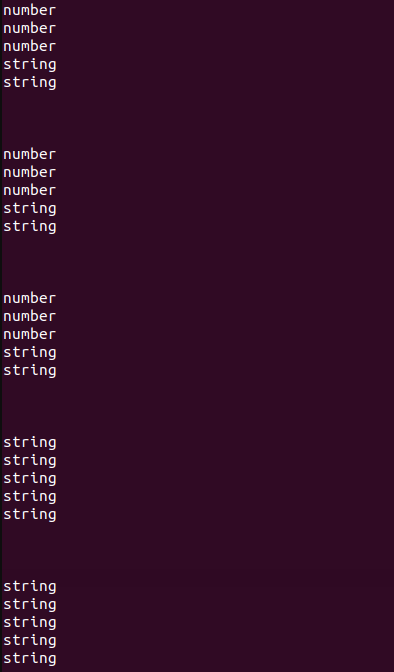Related Articles
Why is [1,2] + [3,4] = “1,23,4” in JavaScript ?
• Last Updated : 02 Mar, 2021

We have given an expression [1, 2] + [3, 4], and the result of this expression is “1,23,4”. In this article, we will know why this weird result we get.

Example :

```=> [1,2] + [3,4]
=> "1,23,4"

=> [1, 2, 3, 4] + [9, 8]
=> "1, 2, 3, 49, 8"

=>  + []
=> "4"

=> [] + []
=> ""```

Before knowing the reason first we understand the addition operator ( + ) and its result with different data types. As we know there are 6 built-in data types in javascript Number, String, Object, Boolean, Undefined and null. And the result of the addition of different kinds of data types is different.

For example: The undefined + undefined => number, undefined + object  => String etc.

In the table below all the result of the addition is given

Example: The example is the way to got that table, by this simple JavaScript program.

## Javascript

 ``

Output : In the above program, there are two arrays in which all 6 data type values are provided. We add every item of arr1 with every item of arr2 and printing the type of the result. That’s how we created the above table.Now come to our problem [1,2] + [3,4], here we are adding two arrays we know that the type of array is an object, so according to the above table when we add two objects then we get the result of string type. JavaScript converts the two arrays into a string and then concatenate them. Here both array becomes “1,2” and “3,4” and when the two strings concatenate then we get “1,23,4”My Personal Notes arrow_drop_up